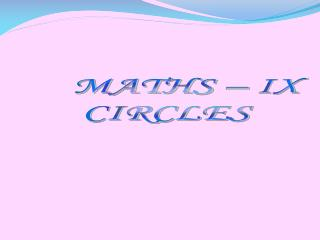# MATHS – IX CIRCLES - PowerPoint PPT PresentationDownload PresentationMATHS – IX CIRCLES

MATHS – IX CIRCLES
Download Presentation## MATHS – IX CIRCLES

- - - - - - - - - - - - - - - - - - - - - - - - - - - E N D - - - - - - - - - - - - - - - - - - - - - - - - - - -
##### Presentation Transcript

1. MATHS – IX CIRCLES

2. CIRCLES Angle Subtended By An Arc Of A Circle

3. If two chords of a circle are equal ,then their corresponding arcs are congruent and conversely ,if two arcs are congruent , then their corresponding chords are equal.(in fig 1.1). D A C FIG 1.1 B

4. Also the angle subtended by an arc at the centre is defined to be angle subtended by the corresponding chord at the centre in the sense that the minor arc subtends the angle and the major arc subtends reflex angle . Therefore , in fig 1.2 ,the angle subtended by the major arc PQ at o is reflex angle POQ .

5. FIG:1.2 O Q P

6. In view of the property above and theorem the following result is true - Congruent arcs (or equal arcs) of a circle subtend equal angles at the centre . Therefore , the angle subtended by a chord of a circle at its centre is equal to the angles subtended by the corresponding (minor) arc at the centre .

7. THEOREM The following theorem gives the relationship between the angles subtended by an arc at a centre and at a point on the circle . THEOREM - The angle subtended by an arc at the centre is double the angle subtended by it at any point on the remaining part of the circle .

8. PROOF Given an arc PQ of a circle subtending angles POQ at the O and PAQ at a point a on the remaining part of the circle . We need to prove that angle POQ = 2 angle PAQ .

9. CASE- (I) A O B Q P FIG:1.3

10. Case -(II) A P o B Q FIG:1.3

11. Case – (III) Q P O B FIG:1.3

12. Consider the three different cases as given in fig 1.3.In (I) , arc PQ is minor , in (II) , arc PQ is a semicircle and in (III) , arc PQ is major. Let us begin by joining AO and extending it to a point B.

13. In all the cases , ^BOQ = ^OAQ + ^AQO Because an exterior angle of a triangle is equal to the sum of the two interior opposite angles.

14. Also in triangle OAQ , OA=OQ (Radii of a circle) Therefore , ^OAQ=^OQA (Theorem) This gives ^BOQ=2^OAQ (I) Similarly , ^BOP=2^OAP (II)

15. From (I) and (II) , ^BOP +^BOQ = 2(^OAP+ ^OAQ) This is same as ^POQ = 2 ^PAQ (III) For the case (III) , where PQ is the major arc , (III) is replaced by reflex angle POQ = 2 ^PAQ.

16. EXAMPLES

17. 1. C If ^AOB =110 degree Then ^ACB =1/2x110 =55 degree. o B A

18. 2 . A C O If^AOB= 40 degree Then ^ACB=1/2x40 =20 degree. B

19. 3. If ^AOB =60 degree Then, The reflex angle of AOB= =360-60 =300 degree. B A O

20. WHAT WE HAVE LEARNT ? If a line segment joining two points subtends equal angles at two other points lying on the same side of the line containing the line segment , the four points lie on a circle . OR The angle subtended by an arc at the centre is double the angle subtended by it at any point on the remaining part of the circle.

21. EXERCISE Question 1. Find the value of^AXB.(AOB=40degree) O X (ii) (i) X O B A B A

22. Answer 1.i If^AOB = 40 degree. Then ^AXB =1/2 x 40 = 20 . Therefore ^AXB =20 degree.

23. If ^AOB = 40 degree Then ^AXB= 2 x 40 = 80. Therefore ^AXB = 80 degree ii

24. ANSWER IN BRIEF • How can the angle subtended by an arc at the centre be defined? • The angle in semicircle is ______ degree. • The angle subtended by an arc at the centre is ______ the angle subtended by it at any point on the circle. • Which is the theorem that defines the relationship between the angles subtended by an arc at a centre and at a point on the circle ? P.S. For answers view the slideshow again